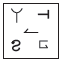# User Forum

Subject :IMO    Class : Class 6

The given question consists of two sets of figures. Figures (i), (ii), (iii) and (iv) constitute the problem set. There is a definite relationship between figures (i) and (ii). Establish a similar relationship between figures (iii) and (iv) by selecting a suitable figure from the options that would replace (?) in figure (iv).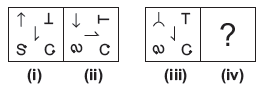(A)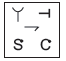(B)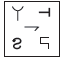(C)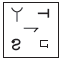(D)